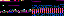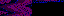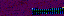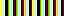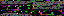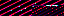```#!/usr/bin/python

from Itemizer import *

if __name__ == "__main__":
Itemizer()
```
```import os
import Itemizer
from Item import *

class Album:

def __init__(self, directory_path, delimiter, copy, simulate, verbosity,
regroup, no_name):
self.set_options(delimiter, copy, simulate, verbosity, regroup, no_name)
self.set_directory_path(directory_path)
self.initialize_item_list()

def set_options(self, delimiter, copy, simulate, verbosity, regroup,
no_name):
self.delimiter = delimiter
self.copy = copy
self.simulate = simulate
self.verbosity = verbosity
self.regroup = regroup
self.no_name = no_name

def set_directory_path(self, directory_path):
if not os.path.isdir(directory_path):
directory_path = None
else:
directory_path = os.path.join(directory_path, "")
self.directory_path = directory_path

def initialize_item_list(self):
self.items = None
if self.directory_path != None:
for file_name in os.listdir(self.directory_path):
path = self.directory_path + file_name
if Itemizer.Itemizer.is_item(path):
number = Itemizer.Itemizer.extract_item_number(path)

if type(paths) == str:
paths = [paths]
current_index = self.build_index(index)
for path in paths:
if not os.path.isfile(path):
else:
if self.items == None:
else:
self.items = self.items.remove_path(path)
if self.items == None:
else:
self.items = self.items.insert(path, current_index)
if current_index:
current_index += 1
if self.verbosity > 1:
print "Added file to list:", path

def build_index(self, index):
if type(index) == str:
index = int(index)
return index

if index == None:
index = 1
self.items = Item(path, index, self.no_name)

def remove(self, paths):
current = self.items
while current != None:
path = self.find_path_in_list(current.path, paths)
if path:
outgoing = current
self.items = self.items.remove_path(outgoing.path)
outgoing.erase_index()
outgoing.save(
self.directory_path, None, self.delimiter, self.copy,
self.simulate, self.verbosity)
paths.remove(path)
current = current.next

def find_path_in_list(self, key, paths):
for path in paths:
if os.path.samefile(key, path):
return path

def commit(self):
if self.directory_path != None and self.items != None:
if self.regroup:
self.items.bunch()
current = self.items
prefix_length = self.determine_prefix_length()
while current != None:
current.save(
self.directory_path, prefix_length, self.delimiter,
self.copy, self.simulate, self.verbosity)
current = current.next

def print_items(self):
current = self.items
while current != None:
print current
current = current.next

def determine_prefix_length(self):
largest_index = self.items.get_largest_index()
return len(str(largest_index))
```
```import os
import re
import shutil

class Item:

def __init__(self, path, index, no_name):
self.path = path
self.index = index
self.no_name = no_name
self.next = None

def __str__(self):
return str(self.index) + "\t" + self.path

def __len__(self):
ii = 0
current = self
while current != None:
ii += 1
current = current.next
return ii

def insert(self, path, index=None):
if index == None:
index = previous.index + 1
item = Item(path, index, self.no_name)
if previous != None:
item.next = previous.next
previous.next = item
else:
item.increase_indices()

previous = None
current = self
while current != None:
if index != None and current.index >= index:
break
previous = current
current = current.next
return previous

def increase_indices(self):
current = self.next
previous_index = self.index
while current != None and current.index == previous_index:
current.index += 1
previous_index += 1
current = current.next

def remove_path(self, path):
previous = None
while current != None:
if os.path.samefile(path, current.path):
if previous != None:
previous.next = current.next
else:
break
previous = current
current = current.next

def bunch(self):
index = 1
current = self
while current != None:
current.index = index
index += 1
current = current.next

def erase_index(self):
self.index = None

def save(self, directory_path, prefix_length, delimiter, copy, simulate,
verbosity):
name = self.extract_name()
name = name.lstrip(delimiter)
prefix = self.build_prefix(prefix_length)
if self.no_name:
file_name = prefix + self.extract_extension(name)
else:
file_name = prefix + delimiter + name
path = os.path.join(directory_path, file_name)
self.write_path(path, copy, simulate, verbosity)

def build_prefix(self, prefix_length):
prefix = ""
if self.index != None:
prefix = str(self.index).zfill(prefix_length)
return prefix

def extract_extension(self, name):
return "." + name.split(".")[-1]

def write_path(self, path, copy, simulate, verbosity):
if not os.path.isfile(path) or not os.path.samefile(self.path, path):
if not simulate:
if copy:
shutil.copy(self.path, path)
else:
shutil.move(self.path, path)
if verbosity > 0:
print "Wrote:", self.path, "=>", path

def get_largest_index(self):
current = self
while current.next:
current = current.next
return current.index

def extract_name(self):
file_name = os.path.basename(self.path)
match = re.match("^[0-9]*(.*)", file_name)
return match.group(1)
```
3.215.182.36
3.215.182.36
3.215.182.36

July 19, 2017

f1. BOSS

Games are corrupt dissolutions of nature modeled on prison, ordering a census from the shadows of a vile casino, splintered into shattered glass, pushing symbols, rusted, stale, charred, ultraviolet harbingers of consumption and violence, badges without merit that host a disease of destruction and decay.

You are trapped. You are so trapped your only recourse of action is to imagine an escape route and deny your existence so fully that your dream world becomes the only reality you know. You are fleeing deeper and deeper into a chasm of self-delusion.

While you're dragging your listless, distending corpus from one cell to another, amassing rewards, upgrades, bonuses, achievements, prizes, add-ons and status boosts in rapid succession, stop to think about what's inside the boxes because each one contains a vacuous, soul-sucking nightmare.

Playing can be an awful experience that spirals one into a void of harm and chaos, one so bad it creates a cycle between the greater and lesser systems, each breaking the other's rules. One may succeed by acting in a way that ruins the world.